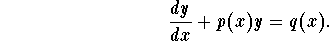# Help solving Homo ODE

• Konig
In summary, the conversation is about solving a first order homogeneous ODE with the equation y'(x)-(a/x)y = b/(x(1+x)^2), where a and b are constants. The attempts so far have involved using an integrating factor x^(-a), but this led to a solution involving Gauss hyper-geometric functions. The conversation also discusses using parts to simplify the integral and comparing the solution to the one reported by Mathematica. The problem is part of a larger project and the individual is experiencing some difficulties with solving it.

#### Konig

Hi, need help solving a first order homogeneous ODE.

y'(x)-(a/x)y = b/(x(1+x)^2) Here a and b are some constants.

Need to solve this for y.

My attempts so far have been to useBut this means solving ∫ x^(-a)/(x(1+x)^2) dx which has solutions in terms of Gauss hyper-geometric functions,

http://en.wikipedia.org/wiki/Hypergeometric_function" [Broken]

Which lead me to believe I'm going wrong somewhere...

Sorry for the maths format, I'm new to here and don't know how to insert LaTeX.

Thanks

Last edited by a moderator:
What, he didn't give you an easy one huh? But isn't the integrating factor x^(-a) so that you get:

$$d(yx^{-a})=\frac{b}{x^{1+a}(1-x)^2}$$

Now suppose all you had to do was:

$$\int \frac{b}{x^{1+a}(1-x)^2}dx$$

Could you use parts say, one, two, three, four times, look at what's happening to the sequence, then come up with a general (infinite-term) expression for the solution that when you checked out the power-series expression for the Hypergeometric series solution reported by Mathematica, the series you get looks like it?

thanks for the response Jackmell,

Am currently working on it, though keep making maths errors which are slowing me down.

I agree it would be a good idea to compare, thanks for the tip.

The problem is part of a project, so yea the problems not meant to be easy.
demoralising thing though is that I'm not sure i was supposed to take this long with it!

Konig